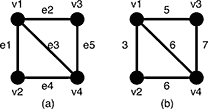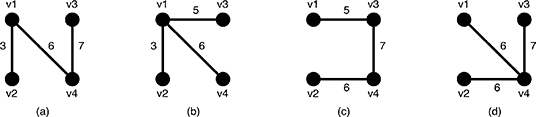# poj 3522 Slim Span（最小差值生成树）

Description

Given an undirected weighted graph G, you should find one of spanning trees specified as follows.

The graph G is an ordered pair (V, E), where V is a set of vertices {v1, v2, …, vn} and E is a set of undirected edges {e1, e2, …, em}. Each edge eE has its weight w(e).

A spanning tree T is a tree (a connected subgraph without cycles) which connects all the n vertices with n − 1 edges. The slimness of a spanning tree T is defined as the difference between the largest weight and the smallest weight among the n − 1 edges of T.Figure 5: A graph G and the weights of the edges

For example, a graph G in Figure 5(a) has four vertices {v1, v2, v3, v4} and five undirected edges {e1, e2, e3, e4, e5}. The weights of the edges are w(e1) = 3, w(e2) = 5, w(e3) = 6, w(e4) = 6, w(e5) = 7 as shown in Figure 5(b).Figure 6: Examples of the spanning trees of G

There are several spanning trees for G. Four of them are depicted in Figure 6(a)~(d). The spanning tree Ta in Figure 6(a) has three edges whose weights are 3, 6 and 7. The largest weight is 7 and the smallest weight is 3 so that the slimness of the tree Ta is 4. The slimnesses of spanning trees Tb, Tc and Td shown in Figure 6(b), (c) and (d) are 3, 2 and 1, respectively. You can easily see the slimness of any other spanning tree is greater than or equal to 1, thus the spanning tree Td in Figure 6(d) is one of the slimmest spanning trees whose slimness is 1.

Your job is to write a program that computes the smallest slimness.

Input

The input consists of multiple datasets, followed by a line containing two zeros separated by a space. Each dataset has the following format.

 n m a1 b1 w1 ⋮ am bm wm

Every input item in a dataset is a non-negative integer. Items in a line are separated by a space. n is the number of the vertices and m the number of the edges. You can assume 2 ≤ n ≤ 100 and 0 ≤ mn(n − 1)/2. ak and bk (k = 1, …, m) are positive integers less than or equal to n, which represent the two vertices vak and vbk connected by the kth edge ek. wk is a positive integer less than or equal to 10000, which indicates the weight of ek. You can assume that the graph G = (V, E) is simple, that is, there are no self-loops (that connect the same vertex) nor parallel edges (that are two or more edges whose both ends are the same two vertices).

Output

For each dataset, if the graph has spanning trees, the smallest slimness among them should be printed. Otherwise, −1 should be printed. An output should not contain extra characters.

Sample Input

Sample Output

# poj 2594 Treasure Exploration（DAG可相交的最小路径覆盖）

Description

Have you ever read any book about treasure exploration? Have you ever see any film about treasure exploration? Have you ever explored treasure? If you never have such experiences, you would never know what fun treasure exploring brings to you.
Recently, a company named EUC (Exploring the Unknown Company) plan to explore an unknown place on Mars, which is considered full of treasure. For fast development of technology and bad environment for human beings, EUC sends some robots to explore the treasure.
To make it easy, we use a graph, which is formed by N points (these N points are numbered from 1 to N), to represent the places to be explored. And some points are connected by one-way road, which means that, through the road, a robot can only move from one end to the other end, but cannot move back. For some unknown reasons, there is no circle in this graph. The robots can be sent to any point from Earth by rockets. After landing, the robot can visit some points through the roads, and it can choose some points, which are on its roads, to explore. You should notice that the roads of two different robots may contain some same point.
For financial reason, EUC wants to use minimal number of robots to explore all the points on Mars.
As an ICPCer, who has excellent programming skill, can your help EUC?

Input

The input will consist of several test cases. For each test case, two integers N (1 <= N <= 500) and M (0 <= M <= 5000) are given in the first line, indicating the number of points and the number of one-way roads in the graph respectively. Each of the following M lines contains two different integers A and B, indicating there is a one-way from A to B (0 < A, B <= N). The input is terminated by a single line with two zeros.

Output

For each test of the input, print a line containing the least robots needed.

Sample Input

Sample Output

floyd求出传递闭包，如果A可以到达B，那么A和B在新图中连边，这样的话在新图中的不相交的最小路径覆盖也就相当于原图的可相交了。

# poj 1422 Air Raid（DAG最小路径覆盖）

Description

Consider a town where all the streets are one-way and each street leads from one intersection to another. It is also known that starting from an intersection and walking through town’s streets you can never reach the same intersection i.e. the town’s streets form no cycles.

With these assumptions your task is to write a program that finds the minimum number of paratroopers that can descend on the town and visit all the intersections of this town in such a way that more than one paratrooper visits no intersection. Each paratrooper lands at an intersection and can visit other intersections following the town streets. There are no restrictions about the starting intersection for each paratrooper.

Input

Your program should read sets of data. The first line of the input file contains the number of the data sets. Each data set specifies the structure of a town and has the format:

no_of_intersections
no_of_streets
S1 E1
S2 E2
……
Sno_of_streets Eno_of_streets

The first line of each data set contains a positive integer no_of_intersections (greater than 0 and less or equal to 120), which is the number of intersections in the town. The second line contains a positive integer no_of_streets, which is the number of streets in the town. The next no_of_streets lines, one for each street in the town, are randomly ordered and represent the town’s streets. The line corresponding to street k (k <= no_of_streets) consists of two positive integers, separated by one blank: Sk (1 <= Sk <= no_of_intersections) – the number of the intersection that is the start of the street, and Ek (1 <= Ek <= no_of_intersections) – the number of the intersection that is the end of the street. Intersections are represented by integers from 1 to no_of_intersections.

There are no blank lines between consecutive sets of data. Input data are correct.

Output

The result of the program is on standard output. For each input data set the program prints on a single line, starting from the beginning of the line, one integer: the minimum number of paratroopers required to visit all the intersections in the town.

Sample Input

Sample Output